### Home > A2C > Chapter 7 > Lesson 7.3.4 > Problem7-202

7-202.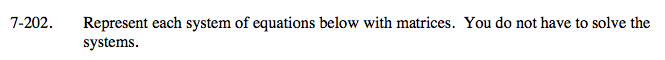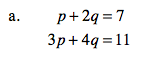$\begin{bmatrix} 1&2\\ 3&4 \end{bmatrix}\begin{bmatrix} p\\ q \end{bmatrix}=\begin{bmatrix} 7\\ 11 \end{bmatrix}$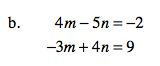See part (a).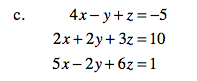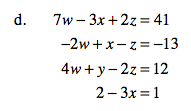See parts (a) and (c); rearranging the equations so the variables are in the same order helps.
Notice that there are four variables in total;filling in 0's for the missing variables is also helpful.